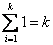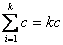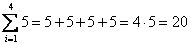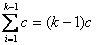## Is there any trick on the Sum of constants?

Yes, there are several tricks. Similar to constant multiplication, because constant do not have index, you can put the constant out of the summation sigma' symbol. Consequently, if you have only summation of a constant, the constant goes out, but one still remain. The summation of one is equal to the total index. Thus,thereforeExampleAs consequent, we can also have the following formula< Previous | Next | Contents >